Courses

# Test: Money - 1

## 10 Questions MCQ Test Mathematics (Maths) for Class 1 | Test: Money - 1

Description
This mock test of Test: Money - 1 for Class 1 helps you for every Class 1 entrance exam. This contains 10 Multiple Choice Questions for Class 1 Test: Money - 1 (mcq) to study with solutions a complete question bank. The solved questions answers in this Test: Money - 1 quiz give you a good mix of easy questions and tough questions. Class 1 students definitely take this Test: Money - 1 exercise for a better result in the exam. You can find other Test: Money - 1 extra questions, long questions & short questions for Class 1 on EduRev as well by searching above.
QUESTION: 1

### Name the coin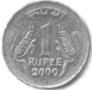Solution: 1 rupee. The answer is correct as we can see the number one (1) engraved on the coin. Below 1 is written rupee in words and the year in which the coin was made. Therefore, as there is 1 rupee written on the coin, the name of the coin is 1 rupee.
QUESTION: 2

Solution:
QUESTION: 3

###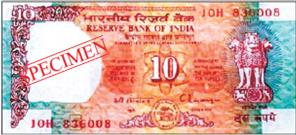Name this note

Solution:
QUESTION: 4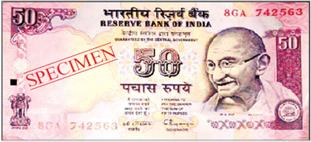Name this note amount.

Solution:
QUESTION: 5

Make 2 rupees by using 1 rupee coins

Solution:
QUESTION: 6

Name the coin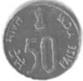Solution:
QUESTION: 7

Name the coin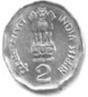Solution: D
QUESTION: 8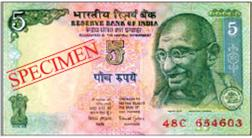Name this note's amount

Solution:
QUESTION: 9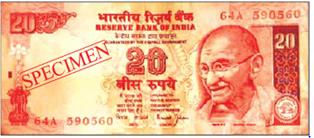Name this note's amount

Solution:
QUESTION: 10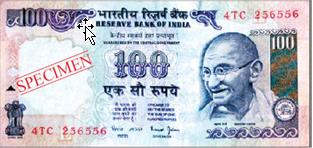Name this note's amount

Solution: